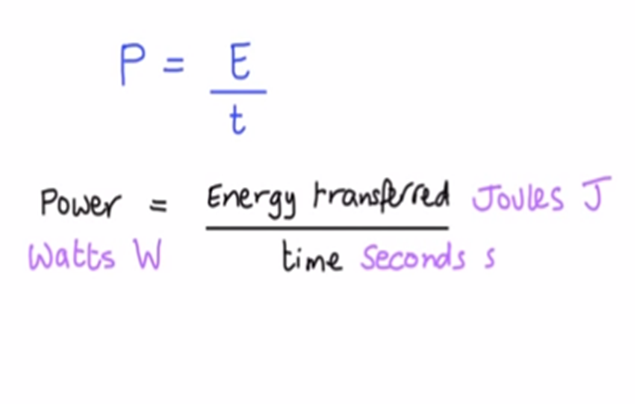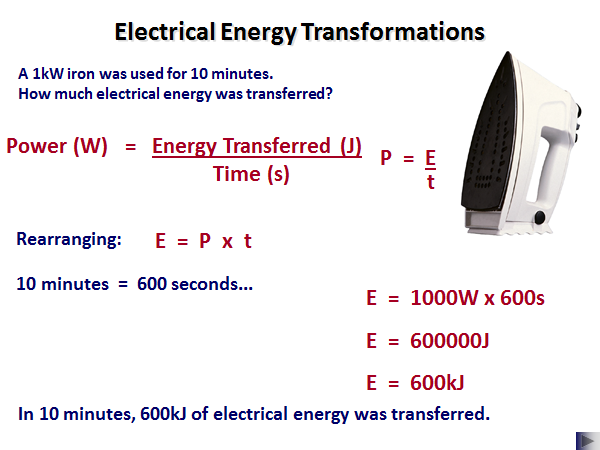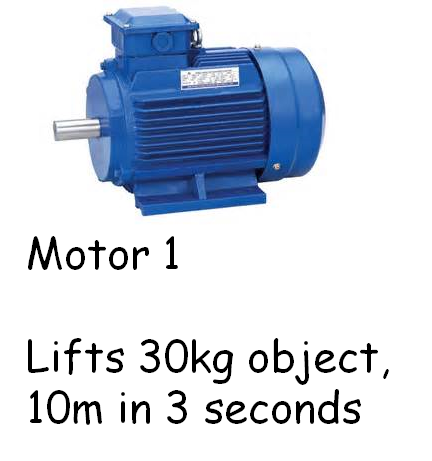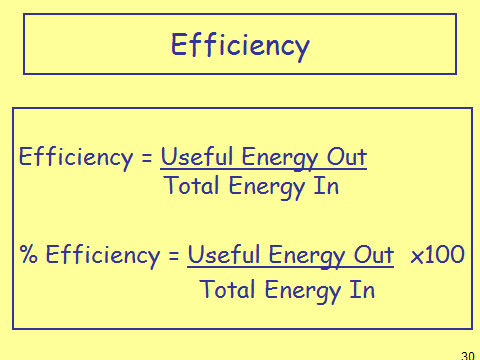# Power

## Power

Power is defined as the rate at which energy is transferred. This means that the higher the power, the quicker energy is transferred.

The equation for power is:This is sometimes written as: P = W/t

In this case W = work done and is also measured in Joules (J).

Example:Notice how the minutes was changed into seconds. For all the physics equations, we must make sure that we use seconds and not minutes or hours.

Also, the 1kW was also converted into 1000W. To convert from kW to W you multiply by 1000.

A more difficult problem: calculate the power of Motor 1.This is an example of a 5 mark question as two equations have to be used.

It is clear from the question that __P = E/t __must be used. We are missing E, energy.

The question include the mass of the object and the height __it is lifted. The only equation that fits these variables is __Ep = m g h

Therefore: Ep = 30 x 10 x 10 = 3000J

We can substitute this value into the power equation

P = 3000/3 = 1000W or 1kW.

## Efficiency

Efficiency is a measure of how well an object outputs useful energy, compared to how much energy is taken in.

The measure is between 0 and 1, where 1 is totally efficient. This can also be measured between 0% and 100% and is done by multiplying your answer by 100.Sometimes you will be asked to leave your answer as a__ decimal__ (first equation) and sometimes as a percentage (second question). It is important to read the question carefully to see which one it wants. If it is unspecified then you can leave your answer in either form.

Sometimes you will be asked how to improve efficiency. We must increase the amount of useful energy and decrease the amount of wasted energy. We can do this a number of ways such as reducing friction or by increasing the amount of insulation around an object or material.

What is the energy supplied to an appliance that is used for 18 hours and has a power usage of 0.1kW?
64800000J
What is the power of an appliance that uses 25J of energy for 5 seconds?
5W
How long does it take to supply a 10W heater with 10kJ of energy?
100s
What is the efficiency of bulb A which has 500J input and 75J of useful output?